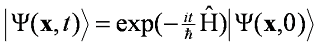## Saturday, 25 October 2008

### TDSE

In a way it is easier to solve the time-dependent Schrödinger equation than the time-independent one. If you don't look too closely at H, it is a first order ordinary differential equation. And not even an eigenvalue problem.If H is constant over time the solution is:You are probably used to this if you have multiplication with a constant. It works the same way with a linear operator like it is the case here.

This way you can propagate a wave packet when you know the potential energy and the wavefunction at the start.

For every Hermitian operator (like H) there is a basis of its eigenfunctions. The physical interpretation is that the state of the system can always be seen as superposition of eigenstates.
Expand Ψ into these functions. And apply the fact that they are eigenfunctions.You have time-independent functions with constant weights, only their phases change. The wave packet is the interference pattern.

The harmonic oscillator is an interesting example. The energies are Ek = (k+1/2)hν. If you plug this in, you will notice that it is a periodic function and the frequency is ν the classical frequency of the oscillator and the frequency of the light that causes the transition. (To be precise: Ψ*Ψ is the same after 2π/ν and Ψ has the opposite sign, it has a period of 4π/ν.) I will show in the next post what such a wave packet looks like.

One more consideration. What happens to an eigenfunction in the time-dependent formulation?It shows a phase change but the physically relevant quantity Ψ*Ψ stays the same. We have a stationary solution.